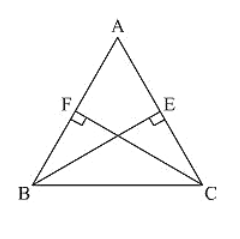# ABC is a triangle in which BE and CF are, respectively,Question:

ABC is a triangle in which BE and CF are, respectively, the perpendiculars to the sides AC and AB. If BE = CF, prove that ΔABC is isosceles

Solution:

Given that ABC is a triangle in which BE and CF are perpendicular to the sides AC and AS respectively such that BE = CF.

To prove, ΔABC is isosceles

Now, consider ΔBCF and ΔCBE,

We have

∠BFC = CEB = 90 [Given]

BC = CB [Common side]

And CF = BE [Given]

So, by RHS congruence criterion, we have

ΔBFC ≅ ΔCEB

Now,

∠FBC = ∠EBC   [Incongruent triangles corresponding parts are equal]

∠ABC = ∠ACB

AC = AB [Opposite sides to equal angles are equal in a triangle]

ΔABC is isosceles